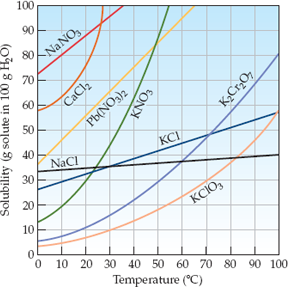×
Get Full Access to Introductory Chemistry - 5 Edition - Chapter 13 - Problem 31p
Get Full Access to Introductory Chemistry - 5 Edition - Chapter 13 - Problem 31p

×

# A solution contains 35 g of NaCl per 100 g of water at 25 °C. Is the solutionISBN: 9780321910295 34

## Solution for problem 31P Chapter 13

Introductory Chemistry | 5th Edition

• Textbook Solutions
• 2901 Step-by-step solutions solved by professors and subject experts
• Get 24/7 help from StudySoup virtual teaching assistantsIntroductory Chemistry | 5th Edition

4 5 1 426 Reviews
18
2
Problem 31P

A solution contains 35 g of NaCl per 100 g of water at 25 °C. Is the solution unsaturated, saturated, or supersaturated? (see Figure 1)

Figure 1 Solubility of some ionic solids as a function of temperatureStep-by-Step Solution:

Solution:

The solution is unsaturated

Explanation:

Step 1

Saturated, means that no more of a given compound can be dissolved in the given solvent. i.e. if something has a solubility of 10 g/L and you have 10 g in a liter, it is saturated.

Supersaturation means going beyond the saturation point. So adding more than is possible. This means, practically, that saturation has been achieved and there is still some solid left in suspension. This often occurs with temperature change, or in a lab situation to ensure saturation.

Unsaturated means that you simply have not added the as much solute as is needed for saturation.

Step 2 of 2

##### ISBN: 9780321910295

The answer to “A solution contains 35 g of NaCl per 100 g of water at 25 °C. Is the solution unsaturated, saturated, or supersaturated? (see Figure 1)Figure 1 Solubility of some ionic solids as a function of temperature” is broken down into a number of easy to follow steps, and 36 words. Introductory Chemistry was written by and is associated to the ISBN: 9780321910295. This full solution covers the following key subjects: figure, solution, solids, ionic, nacl. This expansive textbook survival guide covers 19 chapters, and 2046 solutions. The full step-by-step solution to problem: 31P from chapter: 13 was answered by , our top Chemistry solution expert on 05/06/17, 06:45PM. This textbook survival guide was created for the textbook: Introductory Chemistry, edition: 5. Since the solution to 31P from 13 chapter was answered, more than 929 students have viewed the full step-by-step answer.

Unlock Textbook Solution